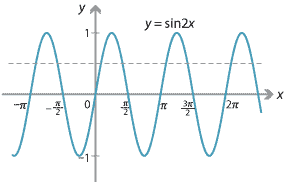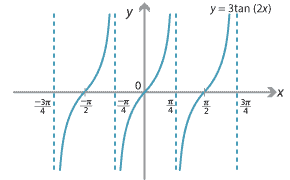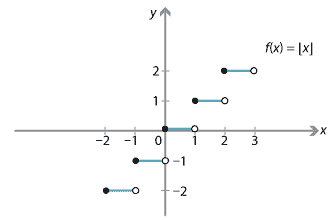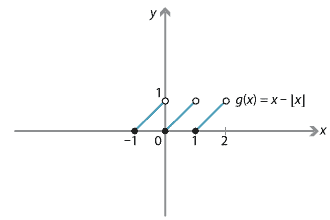##### Exercise 1
• Are 'diagonalise' and 'diagonalize' two words or the same word?
• Is the phrase 'deja vu' two English words? What about 'café'?
• Can we restrict English words to the words defined in the Oxford English Dictionary? Is the word 'gunna' defined? Was 'internet' in the Oxford English Dictionary in 1970?
##### Exercise 2

Let $$f(x) = \dfrac{x^2}{2} + x$$.

1. $$f(3) = \dfrac{3^2}{2} + 3 = \dfrac{15}{2}$$.
2. $$f(a+h) = \dfrac{(a+h)^2}{2} + a + h$$.
##### Exercise 3
1. Let $$f(x) = \sqrt{(x-1)(x-2)}$$. Then $$f(x)$$ is defined if and only if $$(x-1)(x-2) \geq 0$$. So we sketch the graph of $$y=(x-1)(x-2)$$.Hence, $$\mathrm{domain}(f) = (-\infty,1] \cup [2,\infty)$$ and $$\mathrm{range}(f)=[0,\infty)$$.
2. Let $$g(x) = \sqrt{1-2\sin2x}$$. To find the domain of $$g(x)$$, we first note that \begin{align*} g(x) \text{ is defined} \ &\iff\ 1-2\sin2x \geq 0 \\ &\iff\ 2\sin2x \leq 1 \\ &\iff\ \sin2x \leq \tfrac{1}{2}. \end{align*} So we sketch the graph of $$y = \sin 2x$$.Now solve $$\sin2x = \dfrac{1}{2}$$ for $$x$$: \begin{alignat*}{4} 2x &= \dfrac{\pi}{6} + 2k\pi &\quad&\text{or}\quad & 2x &= \dfrac{5\pi}{6} + 2k\pi, &\quad&\text{for some } k \in \mathbb{Z} \\ x &= \dfrac{\pi}{12} + k\pi &&\text{or} & x &= \dfrac{5\pi}{12} + k\pi, &&\text{for some } k \in \mathbb{Z}. \end{alignat*} It follows that $$\sin2x \leq \dfrac{1}{2}$$ if and only if $$x$$ belongs to the interval $$\Bigl[\dfrac{5\pi}{12}, \dfrac{13\pi}{12}\Bigr]$$ or one of its translates by a multiple of $$\pi$$. Hence, $\mathrm{domain}(g) = \bigcup_{k \in \mathbb{Z}}\, \Bigl[\dfrac{5\pi}{12} + k\pi, \dfrac{13\pi}{12}+k\pi\Bigr].$ The maximum value of $$g(x)$$ is $$\sqrt{1 - 2\times (-1)} = \sqrt{3}$$. Hence, $$\mathrm{range}(g) = [0,\sqrt{3}]$$.
##### Exercise 4
Let $$f(x)=3\tan 2x$$. The domain of $$\tan x$$ is all real numbers except odd multiples of $$\dfrac{\pi}{2}$$, and the range of $$\tan x$$ is all reals. Hence, $\mathrm{domain}(f) = \mathbb{R} \setminus \{\dots, -\tfrac{3\pi}{4}, -\tfrac{\pi}{4}, \tfrac{\pi}{4}, \tfrac{3\pi}{4}, \dots\}$ and $$\mathrm{range}(f) = \mathbb{R}$$.Detailed description

##### Exercise 5
1.Detailed description

$$\mathrm{domain}(f) = \mathbb{R}$$ and $$\mathrm{range}(f) = \mathbb{Z}$$.
2.Detailed description

$$\mathrm{domain}(g) = \mathbb{R}$$ and $$\mathrm{range}(g) = [0,1)$$.
##### Exercise 6
1. Arithmetic sequence with first term $$a=2$$ and common difference $$d=3$$.
2. Geometric sequence with first term $$a=3$$ and common ratio $$r=-2$$.
3. Geometric sequence with first term $$a=1$$ and common ratio $$r=0.1$$.
4. The Fibonacci sequence; it is neither geometric nor arithmetic.
5. This sequence is neither geometric nor arithmetic.
##### Exercise 7

$$\mathrm{domain}(f) = \mathrm{codomain}(f) = \mathbb{N}$$ and $$\mathrm{range}(f) = \{3,5,7,9,11,\dots\}$$.

##### Exercise 8

Assume $$A = \{a_1,a_2,\dots,a_m\}$$ and $$B = \{b_1,b_2,\dots,b_n\}$$. If we are defining a function from $$A$$ to $$B$$, then there are $$n$$ choices for where to map $$a_1$$, and then there are $$n$$ choices for where to map $$a_2$$, and so on. Thus the total number of functions is $$n^m$$.